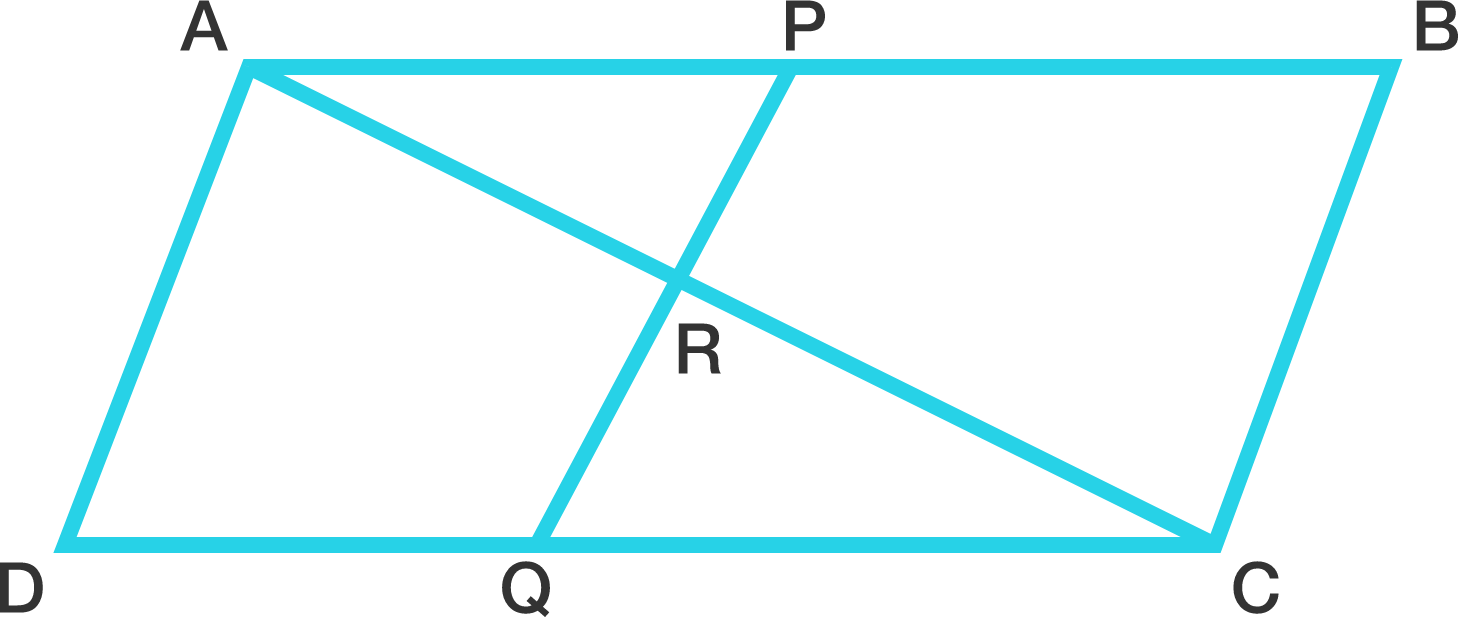# Parallelogram Problem

Geometry Level 5$ABCD$ is a parallelogram. Point $P$ on $AB$ divides it in the ratio $AP : PB = 3 : 2$, and point $Q$ on $CD$ divides it in the ratio $CQ : QD = 7 : 3$. Let $R$ be the intersection of $PQ$ and $AC$. Then, $AR : AC = a : b$, where $a$ and $b$ are positive coprime integers. What is $a + b$?

×